# Representational Similarity Analysis¶

Contributions

Oranges and orange ping-pong balls are not kept next to each other in the grocery store. The oranges are usually placed with other fruits and ping-pong balls with other sport equipment. This helps us shop easily, as we usually group our shopping by categories: fruits and vegetables, meat and dairy, frozen foods, and, somewhere far away, kitchen supplies, toys and sports. Beyond grocery stores, are these meaningful conceptual groupings in the brain? Are patterns of neural activity for oranges and apples more similar to each other as compared to the patterns for oranges and ping-pong balls?

One could conceivably group items based on other attributes such as shape and color. This would make the neural representations of oranges and orange colored ping-pong balls very similar to each other. In a brain region that cares about color, the neural similarity would be greater for oranges and orange ping-pong balls, compared to oranges and red apples. How can we determine the similarity between neural representations and which attributes are driving this similarity?

Representational similarity analysis (RSA) is a way to compare and contrast different brain states and the stimuli that elicited them. In RSA, we compute a similarity measure (often a correlation) between patterns of neural activity for all items being compared. Then, to examine whether neural patterns in a brain region are grouped by color, shape, or category, we can order the similarity measure based on a model that groups by these attributes.

RSA is a highly versatile tool: it can be used to compare brain activity to models, compare data across brain imaging techniques, and even to make cross-species comparisons. You can learn more about the RSA method here and here.

## Goal of this script¶

1. Learn how to perform RSA on a dataset

Calculate and plot Pearson and Spearman correlations in ROIs
Order these similarity matrices in a meaningful way
Interpret a (dis)similarity matrix

2. Visualize similarity with multi dimensional scaling (MDS)

1. Prepare for RSA

2. Create a similarity matrix

3. Manifolds and multi-dimensional scaling (MDS)

Exercises

Novel contribution

### Dataset ¶

The dataset we will be using for this exercise is from Kriegeskorte et al. (2008), called 'Ninetysix' in the datasets folder. 96 visual stimuli, from the 6 categories listed below, were presented to participants. The image stimuli are stored in the subfolder Stimuli.

The data have 6 categories:

1. artificial inanimate (object/scene)
2. human bodypart
3. human face
4. natural inanimate (object/scene)
5. nonhuman bodypart
6. nonhuman face



Self-study: Explore the data

In :
import warnings
import sys
if not sys.warnoptions:
warnings.simplefilter("ignore")

import os
import numpy as np
import pandas as pd
import scipy.io
from scipy import stats
from sklearn.manifold import MDS
import scipy.spatial.distance as sp_distance

import matplotlib.pyplot as plt
import seaborn as sns
from mpl_toolkits.mplot3d import Axes3D

# %matplotlib notebook
%matplotlib inline
%autosave 5
sns.set(style = 'white', context='poster', rc={"lines.linewidth": 2.5})
sns.set(palette="colorblind")

Autosaving every 5 seconds


### 1.1 Load the data for one subject¶

The data for these participants are stored as a matlab file (it was 2008 after all...). Python is able to load matlab files using a scipy utility. However, the file formats can be tricky and may require transformations to make compatible with typical Python organization.

The data from matlab are stored as a dictionary where the variables in the matlab files are keys in the dictionary:

The ROI names are listed in roinames.
The category label indices for each condition are listed in labels.
The label indices correspond to entries in categoryNames.
The data for each ROI, in the order of roinames, is stored in roi_data.

Each row of roi_data represents a stimulus (as defined in labels) and each column represents a voxel (there will be different numbers of voxels in different ROIs). These data have been preprocessed and each entry is stored in terms of t-values. There is no time information and no baseline signal to be used for reference.

Self-study: What do you think these t-values reflect?

The last 4 rows of the dataset have unknown labels (dun dun dunnnnn!). We'll use only the first 92 rows for analysis, for now.

In the analyses that follow we are going to explore the data of subject 'BE'.

### 1.2 Helper Functions ¶

To make it easy for you to achieve the main goals of this notebook, we have created helper functions that extract data from matlab files and convert into numpy arrays.

In :
from utils import load_data_for_a_subj, digitize_rdm, ns_data_dir

In :
# Load the data for one subject
subj_initials = 'BE'
images, roi_data, roi_names, n_rois, categories, n_categories, labels, label_dict = data_pack
n_stimuli = len(images)


Exercise 1: Inspect the data.

• Print the name of each category.
• Report the shape of the data for each ROI and what each dimension means
In :
# Insert code here


### 1.3 Visualize the data ¶

It is always a good idea to plot data as a sanity check before starting analysis.

We also want to see the corresponding labels. Notice the category order is random.

In :
plt.figure()

# Label plot
plt.subplot(2,1,2)
plt.plot(labels)
plt.xlabel('Stimuli', fontsize=16)
plt.ylabel('Category', fontsize=16)

Out:
Text(0, 0.5, 'Category')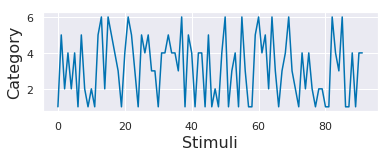Notice that the category order is random i.e. the stimuli at every point are from a different category compared to the neighbors.

## 2. Create a similarity matrix ¶

Let's examine the similarity of the neural representations of each image with the neural patterns of every other image in the dataset. If the neural patterns are similar between images, we will see high values of similarity, but if the neural patterns are dissimilar, we will see low values of similarity.

There are many ways to compute similarity. We start with one of the most common measures of similarity that you are already familiar with: Pearson correlation (see notebook-04). We compute the Pearson correlation on the neural pattern for each image with every other image. We can compute this on data for each of the ROIs that we have just loaded (left and right FFA, and left and right PPA). For each ROI, our computation will result in a 92 x 92 matrix (we only have labels for 92 images). This resulting matrix shows how similar the neural patterns of activity are between images and is called the representational similarity matrix (RSM).

In :
print('ROI names: ', roi_names)

ROI names:  ['lFFA', 'rFFA', 'lPPA', 'rPPA']

In :
# Choose your ROI here!
roi_id = 1

# Plot figure of these correlations
f, ax = plt.subplots(1,1, figsize=(8, 7))

plt.imshow(
np.corrcoef(roi_data[roi_id]),
cmap='bwr',
vmin=-1,
vmax=1,
)
plt.colorbar()
ax.set_title('RSM, unsorted, %s' % (roi_names[roi_id]))
ax.set_xlabel('stimuli id')
ax.set_ylabel('stimuli id')

Out:
Text(0, 0.5, 'stimuli id')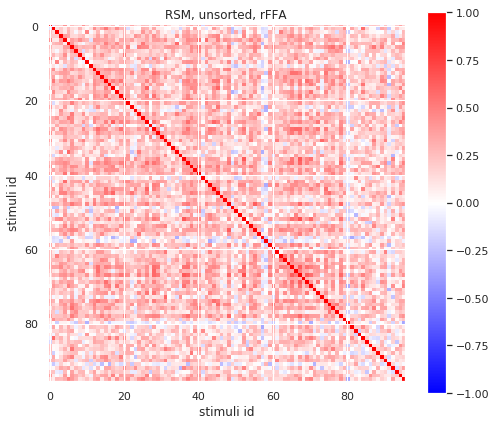Exercise 2: In the plot above you used Pearson correlation to compute similarity. An alternative metric is a Spearman correlation.

• Explain the difference between Pearson and Spearman correlation.
• Redo the analysis above with Spearman correlation.
• Visualize the RSM based on Spearman correlation.

A:

In :
# Insert code here


### 2.1 Reorder data into categories ¶

Although the plot above is useful, it is hard to observe any structure because the order of the stimuli is random. To simplify, let's reorganize into label groups.

In :
# Add the stimulus condition labels so that we can sort the data, collecting rows from the same condition together.
sort_ids = labels.argsort()
lffa_sorted = roi_data[sort_ids, :]

plt.figure(figsize=(9,7))

# Plot the new sorted results
plt.subplot(2,1,1)
plt.plot(lffa_sorted[:,0])
plt.ylabel('t-Value', fontsize=16)
plt.xlim(0, 96)

plt.subplot(2,1,2)
plt.plot(labels[sort_ids])
plt.xlabel('Stimuli', fontsize=16)
plt.ylabel('Category', fontsize=16)
plt.show()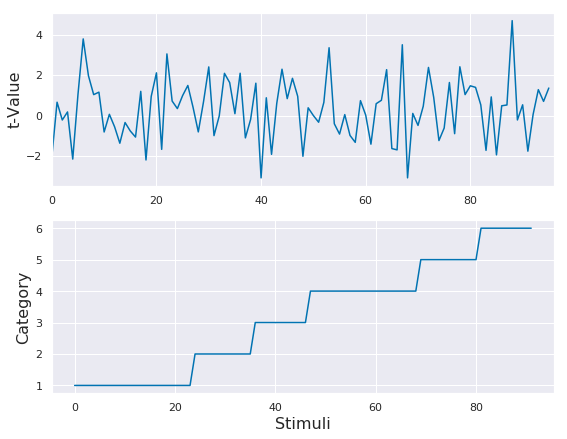In :
# Choose your ROI here!
roi_id = 1

# Calculate the RSM
rsm = np.corrcoef(roi_data[roi_id][sort_ids, :][:92,])

# Plot
f, ax = plt.subplots(1,1, figsize=(10, 8))
plt.imshow(
rsm,
cmap='bwr',
vmin=-1,
vmax=1,
)
plt.colorbar()

# Pull out the bin edges between the different categories
binsize = np.histogram(labels[:92,], 6)
edges = np.concatenate([np.asarray(), np.cumsum(binsize)])[:-1]
ax.set_xticks(list(np.array(edges)+8))
ax.set_xticklabels(categories, rotation = 30)
ax.set_yticks(list(np.array(edges)+8))
ax.set_yticklabels(categories)
ax.vlines(edges,0,92)
ax.hlines(edges,0,92)
ax.set_title('RSM, sorted, %s' % roi_names[roi_id])

Out:
Text(0.5, 1.0, 'RSM, sorted, rFFA')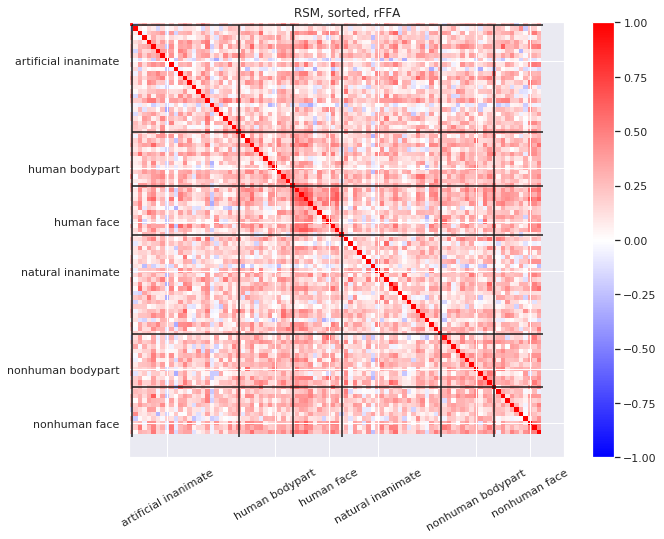Binning the data: In Figure 1 of Kriegeskorte et al. (2008), the raw correlation values were binned into ten bins based on the percentile score of the dissimilarity value, and the percentile value was plotted. We have created a function digitize_rdm to perform the same calculation here and make the plots similar to Figure 1 in Kriegeskorte et al. (2008).

Note that Figure 1 was using dissimilarity rather than similarity and that the data were from a different ROI (inferior temporal cortex, or IT). However, we can apply the same function to our RSM, the only difference being that the percentile will be based on similarity.

The digitize_rdm functions works in the following manner:

1. Create n_bins of percentile values.
2. Take in the matrix of correlations and reshape it into a single row.
3. Determine the percentile value of every correlation point and assign it to a bin (np.digitize does this).
4. Reshape the assigned percentile values into the original correlation matrix shape.
5. Finally, plot the percentile values.

In :
# Plot the RSM
f, ax = plt.subplots(1,1, figsize=(10, 8))
plt.imshow(
digitize_rdm(rsm), cmap='jet',
)
plt.colorbar()

# Pull out the bin edges between the different categories
binsize = np.histogram(labels[:92,], 6)
edges = np.concatenate([np.asarray(), np.cumsum(binsize)])[:-1]
ax.set_xticks(list(np.array(edges)+8))
ax.set_xticklabels(categories, rotation = 30)
ax.set_yticks(list(np.array(edges)+8))
ax.set_yticklabels(categories)
ax.vlines(edges,0,92)
ax.hlines(edges,0,92)
ax.set_title('RSM digitized %s' % roi_names[roi_id]);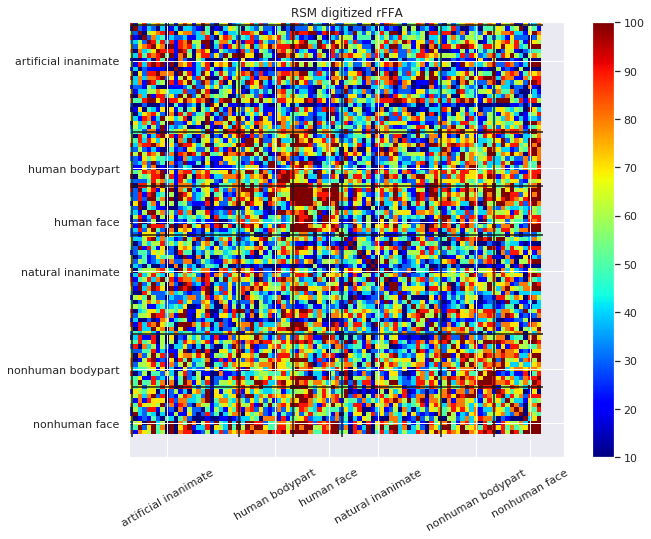In [ ]:



Exercise 3: This new organization is helpful but could be improved (based on our knowledge of the brain). Order the datapoints so that the categories are as follows: human face, human body part, non-human face, non-human body part, natural inanimate and artificial inanimate. This will make for a nicer looking correlation matrix and will help you see any structure within and between categories.

• Write a function to re-order the data.
• Recompute the RSM based on the re-ordered data and visualize it.
• Visualize the digitized RSM using the digitization function provided earlier.
In :
# Insert code here


### 2.2 How to read a similarity matrix ¶

It is important to be able to read a similarity matrix at a glance. There are couple features to look out for and we will highlight these with some toy data.

Imagine a scenario where we have 12 trial types (e.g., images) grouped into 4 categories (e.g., faces, objects, body parts, and scenes). We are going to simulate some data that has similar activity within category but different activity between categories.

#### 2.2.1. Create simulated data.¶

In :
trial_types = 12
n_sim_categories = 4
repetitions_per_trial = 24
trial_noise = 0.5 # multiplying factor for the noise for each trial.
trials_per_category = int(trial_types / n_sim_categories)

# The template per category. One common signal per category.
# This will be the similarity within category.
category_templates = np.random.randn(n_sim_categories, repetitions_per_trial)

# Add some noise to each trial and add the category template to each trial.
# This will create the trial activity.
trials = np.zeros((trial_types, repetitions_per_trial))
for category_counter in range(n_sim_categories):
category_trials = np.random.randn(trials_per_category, repetitions_per_trial) * trial_noise
for trial_counter in range(trials_per_category):
trials[(trials_per_category * category_counter) + trial_counter, :] = category_templates[category_counter, :] + category_trials[trial_counter, :]


#### 2.2.2. Compute correlation and plot the result¶

In :
# Create the correlation matrix
sim_r1 = np.corrcoef(trials)
plt.figure(figsize=(8, 8))
plt.imshow(sim_r1,
interpolation='none',
cmap='bwr',
vmin=-1,
vmax=1,
)
plt.colorbar()

Out:
<matplotlib.colorbar.Colorbar at 0x7f762efb9128>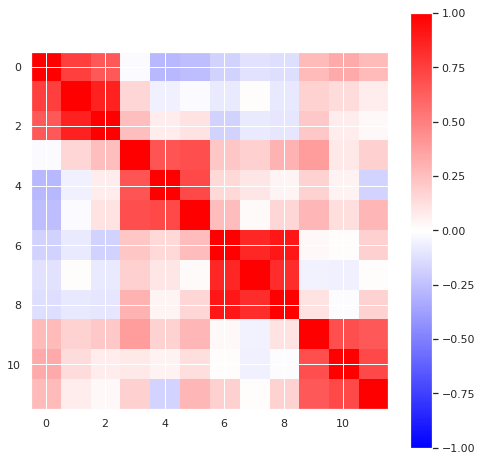In the above plot you should see some clear blocking structure along the diagonal: items within a category are more similar to one another than they are to items in different categories.

#### 2.2.3. Make two categories similar and observe changes in the similarity matrix¶

Below we create a plot where there is off-diagonal structure. High similarity in off-diagonal parts of a similarity matrix means that elements that are far apart in the ordering have similar structure. In this toy simulation we create an example where the first and third categories are similar to one another (i.e., faces and body parts).

In :
# Overwrite the template for the 3rd category with the template for 1st category.
# Python indexing begins at .
category_templates[2, :] = category_templates[0, :]

# Create the trial activity
trials = np.zeros((trial_types, repetitions_per_trial))
for category_counter in range(n_sim_categories):
category_trials = np.random.randn(trials_per_category, repetitions_per_trial) * trial_noise
for trial_counter in range(trials_per_category):
trials[(trials_per_category * category_counter) + trial_counter, :] = category_templates[category_counter, :] + category_trials[trial_counter, :]

# Create the correlation matrix
sim_r2 = np.corrcoef(trials)
plt.figure(figsize=(8, 8))
plt.imshow(sim_r2,
interpolation='none',
cmap='bwr',
vmin=-1,
vmax=1,
)
plt.colorbar()

Out:
<matplotlib.colorbar.Colorbar at 0x7f762eed9630>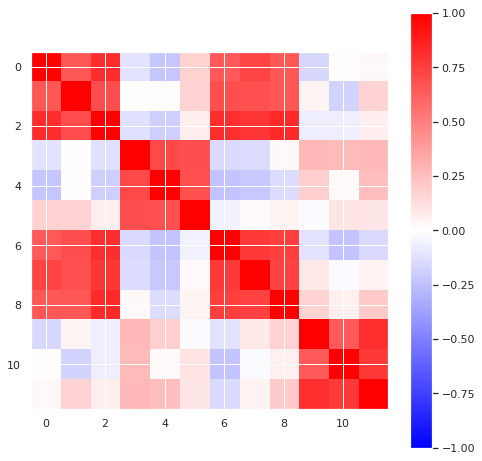Exercise 4: Now use your new knowledge about reading a similarity matrix to interpret the matrix you created for the real data Exercise 3.

A:

### 2.3 Representational dissimilarity ¶

The previous analyses framed everything in terms of similarity between the items. However people sometimes prefer to consider this type of data in terms of dissimilarity. This close cousin of the similarity matrix is called the representational dissimilarity matrix (RDM). The dissimilarity matrix is computed simply as 1 - correlation.

Exercise 5: Plot the RDM for the right FFA ROI using the new order as you created in Exercise 3.

In :
# Insert code here


Exercise 6: For RDM plots based on correlation values, what does an RDM value greater than 1 correspond to in terms of a correlation?

A:

### 2.4 Comparison of representations in monkeys and humans ¶

The RSA can be used to compare information not just in humans, but across species too. Below is comparison of the RDM for monkeys and humans, in the inferior temporal cortex (Color map altered to match Figure 1 in Kriegeskorte et al. (2008)).

In :
# Load the data, and bin to percentile

monkey_rdm_digitized = digitize_rdm(monkeyRDM)
human_rdm_digitized = digitize_rdm(humanRDM)

f, axes = plt.subplots(1,2, figsize = (14, 6))
axes.imshow(
monkey_rdm_digitized,
cmap='jet',
)
axes.imshow(
human_rdm_digitized,
cmap='jet',
)
# plt.colorbar()
axes.set_title('Monkey RDM')
axes.set_title('Human RDM')

#for i in range(2):
#    axes[i].set_xlabel('stimuli id')
#    axes[i].set_ylabel('stimuli id')

Out:
Text(0.5, 1.0, 'Human RDM')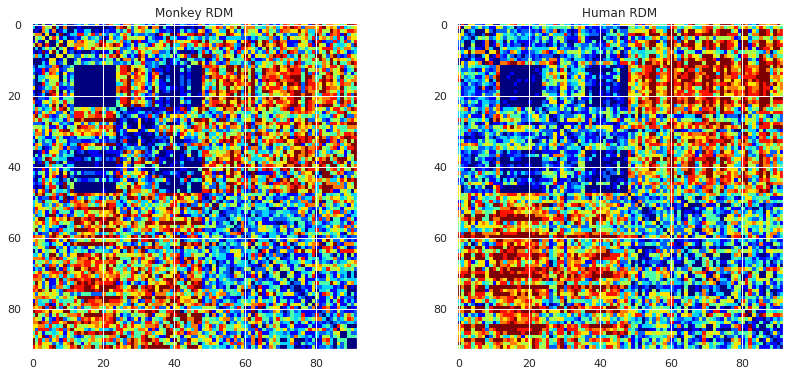## 3. Multi-dimensional scaling (MDS) ¶

The correlation matrix for the 92 images describes how similar each item is to each other item. This means that if two items have a high positive correlation then they can be thought of as eliciting a very similar activation pattern across voxels. We can reframe this to be thought of as a distance in a high-dimensional space. From this perspective, items that are similar to one another will be grouped close together and far away from points that they are dissimilar to.

MDS allows us to visualize the similarity of our data in a different way than plotting the matrices above. Specifically, it allows to generate a lower-dimensional image (e.g., 2-D or 3-D) in which the distances between points approximate the distances in the original high-dimensional data. There is an MDS method built into scikit-learn.

In :
# Create a 2-D MDS
mds = MDS(n_components=2, dissimilarity="precomputed", random_state=0)  # Create the MDS object
results = mds.fit(digitize_rdm(1 - rsm))  # Use the dissimilarity matrix


Exercise 7: How does changing the order of the data (e.g., shuffling the rows/columns) in your RDM affect the distance between points calculated by MDS?

A:

### 3.1 Plot the 2D structure of the RDM ¶

We'll plot the 92 images on a "map" signifying how close or far apart images are to each other. We use different colors for the 6 categories of images.

In :
coords = results.embedding_

plt.figure(figsize=(10, 7))
for label_counter in np.unique(labels[:92]):
label_idxs = (labels[:92] == label_counter)[:]
plt.scatter(
coords[label_idxs, 0], coords[label_idxs, 1],
marker = 'o', s = 50
)
plt.legend(categories, bbox_to_anchor=(1, .8), loc="upper left")
plt.title('MDS, 2D');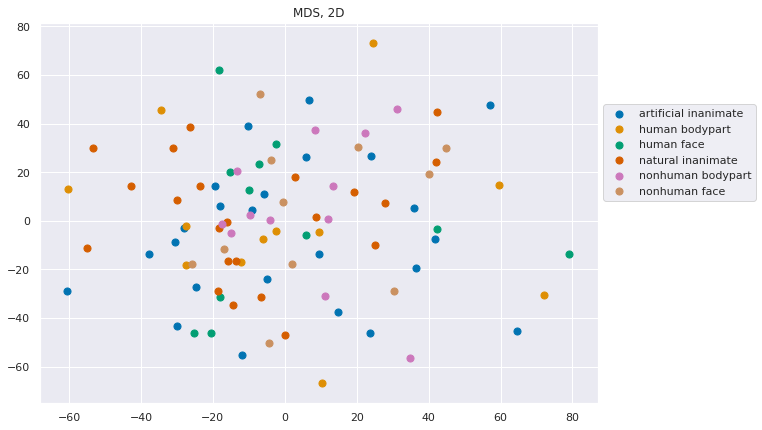Self-study: On the MDS plot you are currently plotting each item as a point. You could instead load in each image and plot that image on the MDS plot directly to get a feel for which stimuli are being grouped.

### 3.2 Plot the 3D structure of the RDM ¶

MDS is just trying to find a k-dimensional embedding that minimizes the stress (something akin to the goodness of fit). This means we can actually plot it in arbitrarily high dimensions to try and capture the data structure. Below we make a 3D plot, given that the 2D plot.

In :
# Multi-dimensional scaling
mds = MDS(n_components=3, dissimilarity="precomputed", random_state=0)
results = mds.fit(digitize_rdm(1 - rsm))

coords = results.embedding_

fig = plt.figure(figsize=(10, 7))
for label_counter in np.unique(labels[:92]):
label_idxs = (labels[:92] == label_counter)[:]
ax.scatter(
coords[label_idxs, 0], coords[label_idxs, 1], coords[label_idxs, 2],
marker = 'o', s = 50
)

plt.legend(categories, bbox_to_anchor=(1,.7), loc="upper left")
plt.title('MDS, 3D')
plt.tight_layout()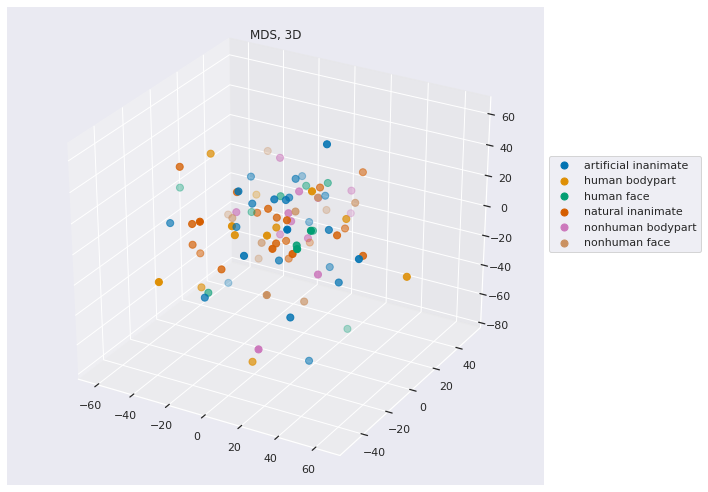There are tools available to us to evaluate what is the appropriate dimensionality for visualization of our data (i.e. what dimensionality has sufficiently low stress). We can look at the stress of the MDS with different numbers of components (i.e. different dimensionality) and determine what dimensionality of the data is appropriate.

Let's make a toy problem to start off with in order to get a handle on what this should look like. We are going to make points that lie on a line in 3D space. Because a line has only one dimension of information (along its length) the data ought to be able to be reduced in dimensionality to a single dimension. We will run MDS on this data to see if that is the case.

In :
coords = np.linspace(1, 30, 30)
coords = np.vstack((coords, coords, coords)).T

fig = plt.figure()
ax.scatter(coords[:, 0], coords[:, 1], coords[:, 2])

Out:
<mpl_toolkits.mplot3d.art3d.Path3DCollection at 0x7f762e4a3e80>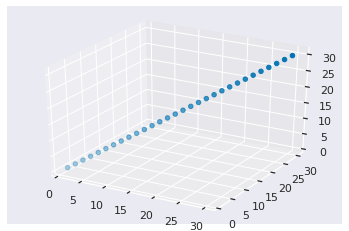In :
# Calculate the euclidean distance of every point from every other point
dist = sp_distance.squareform(sp_distance.pdist(coords))

# Iterate through different numbers of components
stress = []
for components in range(1, 6):
mds = MDS(n_components=components, dissimilarity="precomputed", random_state=0)
# Pull out the stress of the MDS fit
stress.append(mds.fit(dist).stress_)

# Plot the stress
plt.figure();
plt.plot(range(1, 6), stress)
plt.ylabel('Stress')
plt.xlabel('Dimensionality')

Out:
Text(0.5, 0, 'Dimensionality')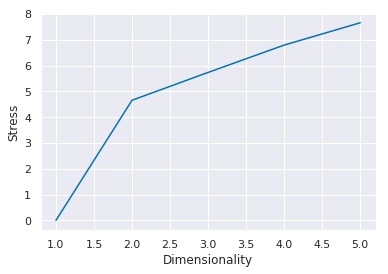Exercise 8: It is not typical for data to be able to be described accurately in low dimensionality: stress generally decreases with the number of components. Perform a similar analysis below to estimate the appropriate dimensionality to visualize the RDM of the right FFA data from this participant. What is the appropriate lower dimensional representation of the data? Note: Make sure you don't calculate the stress metric from the MDS embedding, calculate the MDS fit from the RDM.

Here's the list of arguments for MDS:

MDS(n_components=2, metric=True, n_init=4, max_iter=300, verbose=0, eps=0.001, n_jobs=None, random_state=None, dissimilarity=’euclidean’)

Empirically, more stringent convergence criteria (i.e. large n_init and max_iter, small eps) will lead to more stable results.

A:

In :
# Insert code here


Exercise 9: Compute RDMs and create MDS plots for the left PPA and right PPA using the reordering you created above.

In :
# Insert code here


Exercise 10: The last four rows in the dataset for subject BE have unmarked labels. One of them is a human face. Using the techniques outlined here and your knowledge of the category preference of each ROI, analyze the data to make a best guess of which one of the 4 missing labels is a human face. Show your work and reasoning that led you to this conclusion. Hint: It will help to visualize these 4 points amongst the points you already have. It would also help to compare the response of each of the missing data points with a 'canonical' face response.

In :
# Insert code here


Novel contribution: be creative and make one new discovery by adding an analysis, visualization, or optimization.

Some examples:

• visualize the average RDM across subjects
• compare the empirical RDM to some theoretical RDM
• check the consistency between hierarchical clustering vs. ground truth label ordering
• use other dimensionality reduction methods to visualize the data (PCA, tSNE, etc.)
• perform some classification on this data set
• apply RSA on previous datasets (e.g. VDC, the simulated dataset used in the 1st notebook)
• apply RSA on previous datasets (e.g., VDC)
• even better, your own ambitious ideas!

## Contributions ¶

M. Kumar, C. Ellis and N. Turk-Browne produced the initial notebook. 02/2018
The mystery label exercise was borrowed from a matlab version created by F. Pereira.
T. Meissner minor edits.
Q. Lu plot aesthetics, digitization func, modularize funcs, re-ordering, replicate the original paper.
K.A. Norman provided suggestions on the overall content and made edits to this notebook.
C. Ellis implemented updates from cmhn-s19